# Graphs in Science Graphs in Science What type

• Slides: 25
Download presentationGraphs in ScienceGraphs in Science What type of data can line graphs display? How do you determine a line of best fit or the slope of a graph? Why are line graphs powerful tools in science?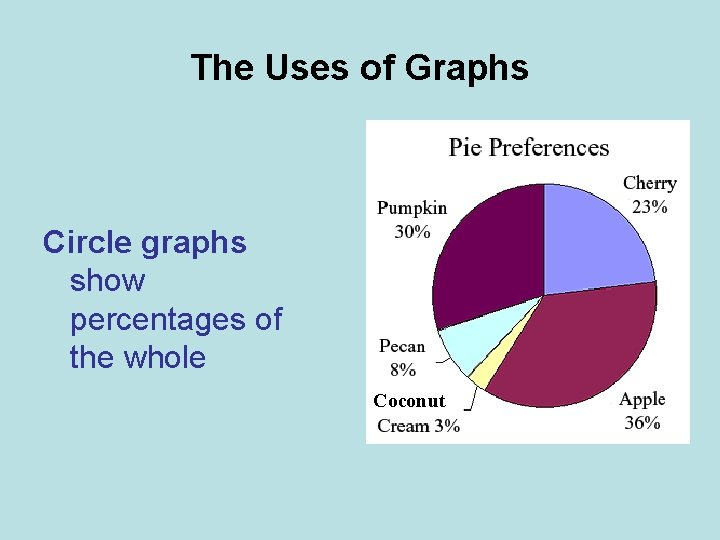The Uses of Graphs Circle graphs show percentages of the whole Coconut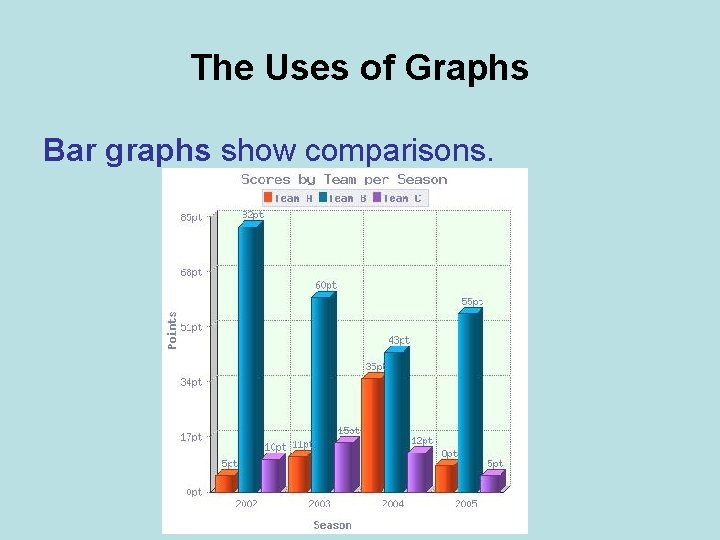The Uses of Graphs Bar graphs show comparisons.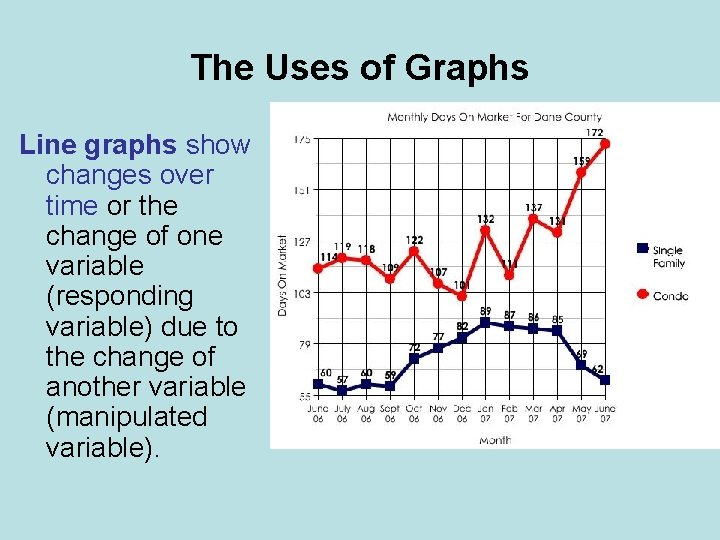The Uses of Graphs Line graphs show changes over time or the change of one variable (responding variable) due to the change of another variable (manipulated variable).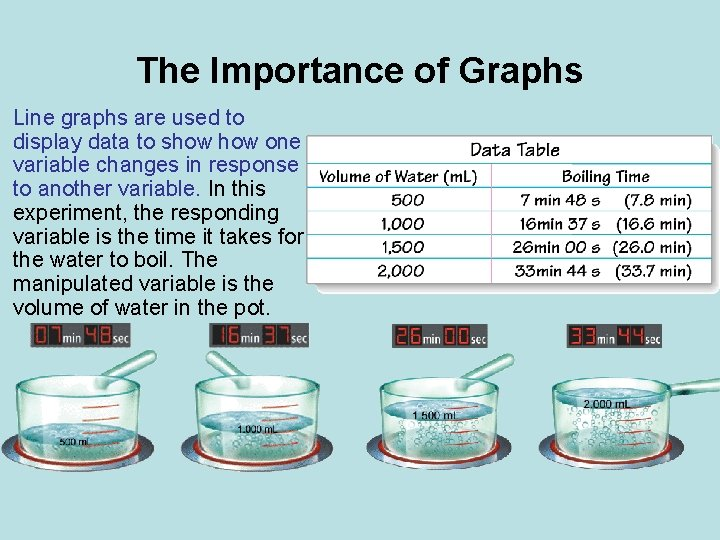The Importance of Graphs Line graphs are used to display data to show one variable changes in response to another variable. In this experiment, the responding variable is the time it takes for the water to boil. The manipulated variable is the volume of water in the pot.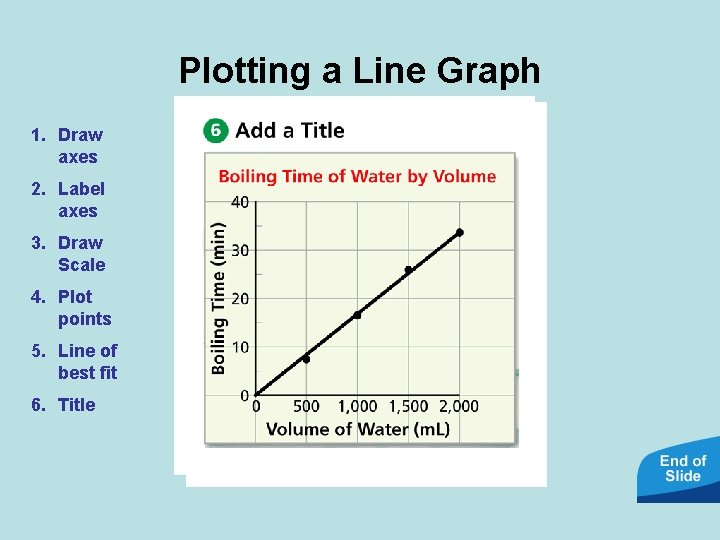Plotting a Line Graph 1. Draw axes 2. Label axes 3. Draw Scale 4. Plot points 5. Line of best fit 6. TitleWhy Draw a Line of Best Fit? A line of best fit emphasizes the overall trend shown by all the data taken as a whole.Slope (Rate of Change) The slope (rate of change) of a graph line tells you how much y changes for every change in x.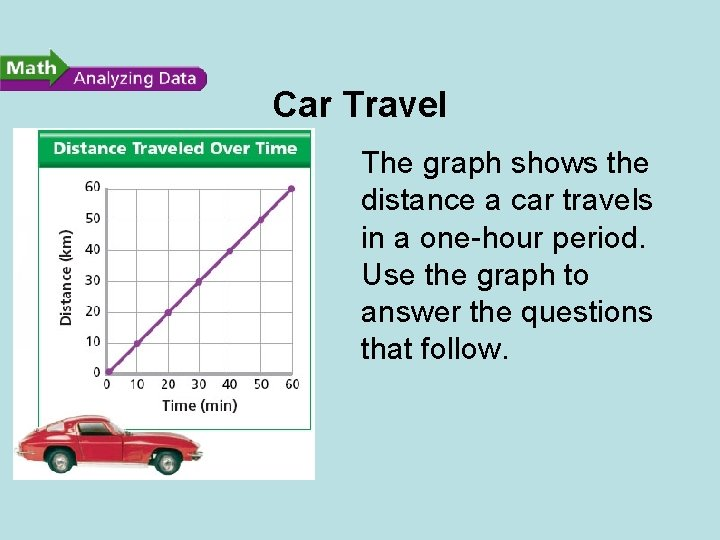Car Travel The graph shows the distance a car travels in a one-hour period. Use the graph to answer the questions that follow.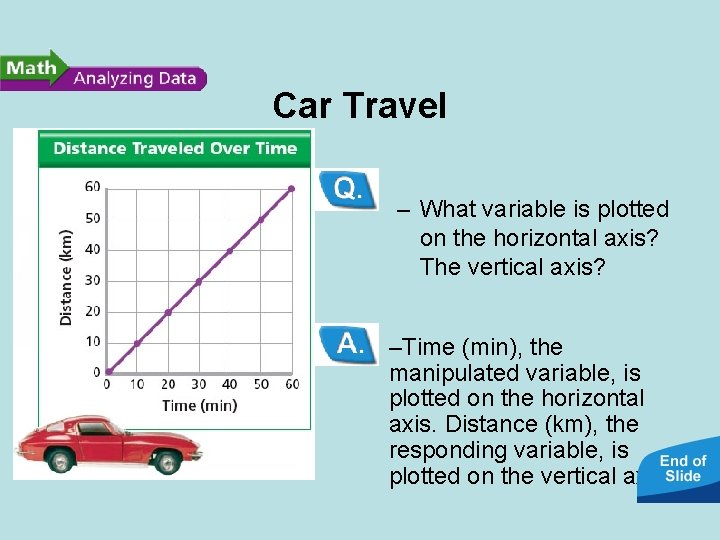Car Travel • Reading Graphs: – What variable is plotted on the horizontal axis? The vertical axis? –Time (min), the manipulated variable, is plotted on the horizontal axis. Distance (km), the responding variable, is plotted on the vertical axis.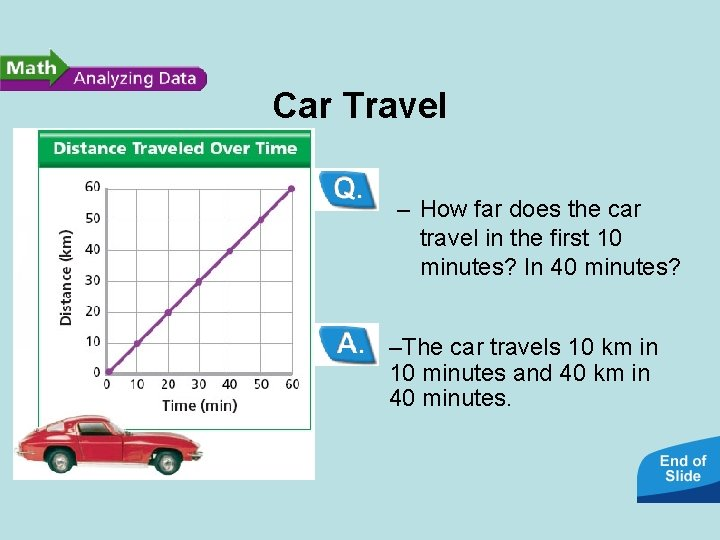Car Travel • Interpreting Data: – How far does the car travel in the first 10 minutes? In 40 minutes? –The car travels 10 km in 10 minutes and 40 km in 40 minutes.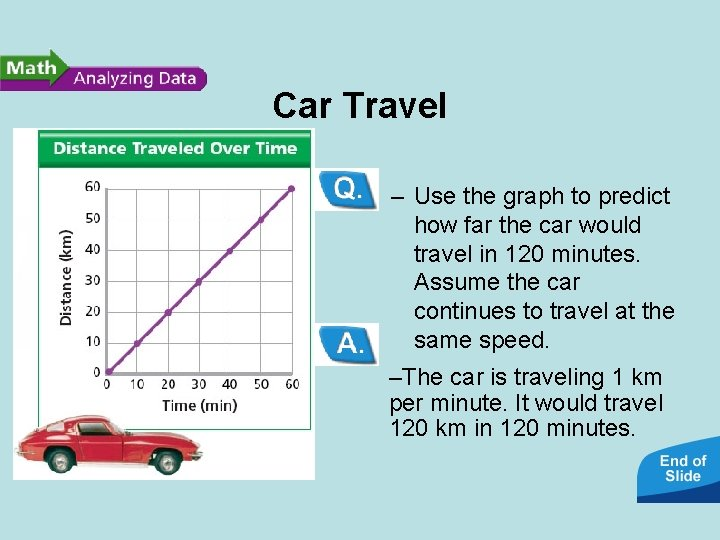Car Travel • Predicting: – Use the graph to predict how far the car would travel in 120 minutes. Assume the car continues to travel at the same speed. –The car is traveling 1 km per minute. It would travel 120 km in 120 minutes.Car Travel • Calculating: – Calculate the slope (rate of change) of the graph. What information does the slope provide? –The slope (rate of change) is 1 km/min. The slope (rate of change) provides information about the car’s average speed.Using Graphs to Identify Trends Line graphs are powerful tools in science because they allow you to identify trends and make predictions.Using Graphs to Identify Trends Line graphs are powerful tools in science because they allow you to identify trends and make predictions.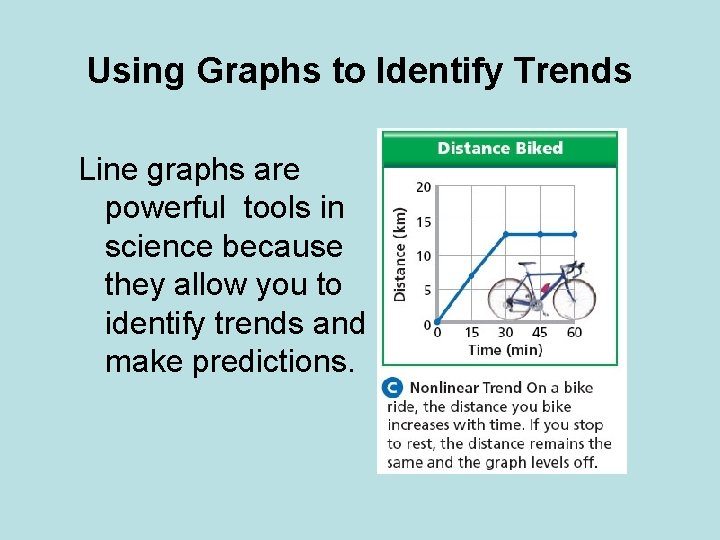Using Graphs to Identify Trends Line graphs are powerful tools in science because they allow you to identify trends and make predictions.Using Graphs to Identify Trends Line graphs are powerful tools in science because they allow you to identify trends and make predictions.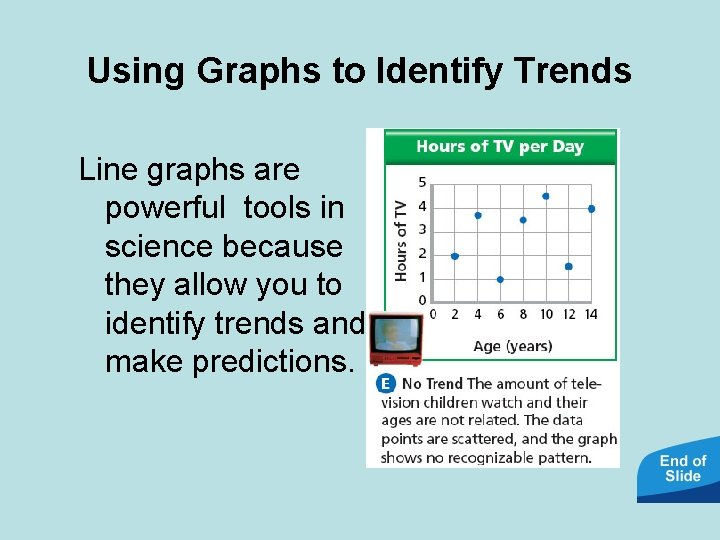Using Graphs to Identify Trends Line graphs are powerful tools in science because they allow you to identify trends and make predictions.• https: //www. youtube. com/watch? v=r 0 F 7 dr Dbf 9 I• What would you use if you were comparing how many miles a car had to how old it was, what type of graph would you use?• If you wanted to create a graph to show the amounts of ingredients in a certain food, what graph would you use?• If we wanted to show amounts of food eaten by a person in a given period of time for several people, what graph would we use?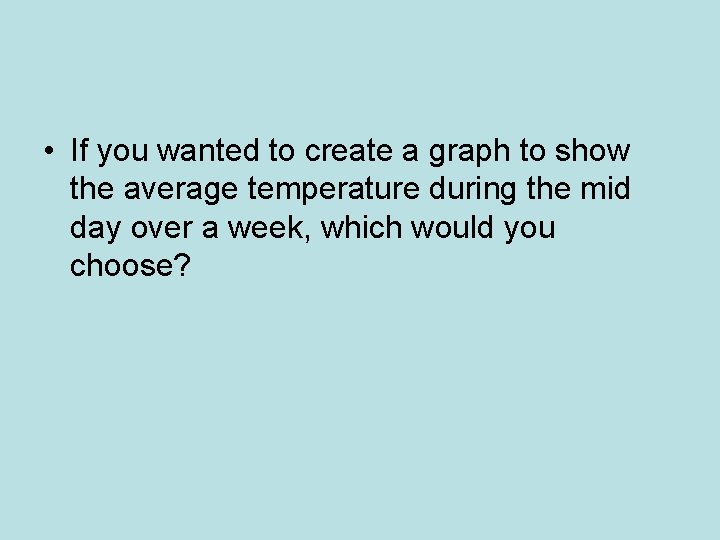• If you wanted to create a graph to show the average temperature during the mid day over a week, which would you choose?